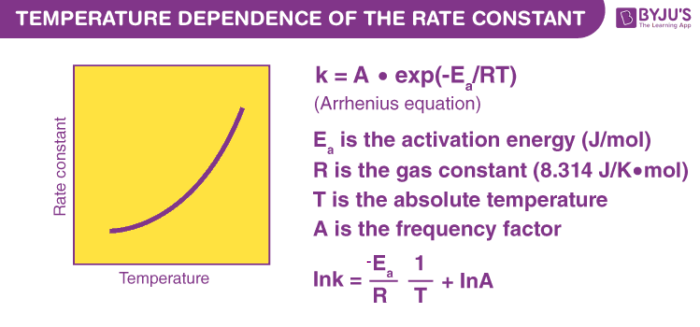# Effect of Temperature on Rate of Reaction

Reaction Kinetics deals with the Study of Rates of Chemical Reactions or Processes. It is one of the most advanced studies in the field of physical chemistry. The information about these factors affecting the rate of a chemical reaction help engineers and scientists to economically scale up the reactions to industrial scale in various industries. It is therefore very important for us to know how the rate of a chemical reaction gets affected.

## Effect of Temperature

Temperature is one of the parameters that can affect the rate of a chemical reaction considerably. We have often seen milk boiling on a gas stove. The rate at which a specific quantity of milk boils depends on the flame of the stove. If the flame height is maximum, the milk boils in less time and if the flame height is minimum, the milk takes more time to boil. Here the flame height resembles temperature.If the temperature is high, the milk attains its boiling point in less time and if the temperature is low, the milk takes more time to attain its boiling point. The boiling of milk is not the only reaction that gets affected by temperature. Most of the chemical reactions show a change in their reaction rate with the varying in temperature.

It has been observed that the rate constant for a chemical reaction gets doubled for every 10oC rise in temperature. Until 1889, there was no fixed way to physically measure the temperature dependence of the rate of a chemical reaction. In 1889, Svante Arrhenius extended the work of J.H van’t Hoff and proposed an equation that related temperature and the rate constant for a reaction quantitatively. The proposed equation was named as Arrhenius Equation.

## Arrhenius Equation

Here is the Arrhenius Equation on the temperature dependence of the rate of a chemical reaction.

$$k = A e^{\frac {-E_a}{RT}}$$

Where,

k= rate constant of the reaction
A= Arrhenius Constant
Ea= Activation Energy for the reaction (in Joules mol−1)
R= Universal Gas Constant
T= Temperature in absolute scale (in kelvins)

We knew that the rates of reaction increase with an increase in temperature but it was not known on how to predict the relation between temperature and reaction rates quantitatively. Arrhenius Equation has enabled us to overcome this problem. It is an empirical relation that is used to model the variation of the rate constant with the temperature, that in turn gives information about the rates of chemical reactions at different temperatures.

## Frequently Asked Questions – FAQs

### What is rate of reaction in chemistry?

In chemistry, reaction rate is the frequency at which a chemical reaction proceeds. It is also expressed either in terms of the concentration (amount per unit volume) of a substance produced in a time unit, or the concentration of a reactant absorbed in a time unit.

### What is the SI unit of reaction rate?

Usually reaction rates are expressed as the reactant concentration consumed or the product concentration produced per unit time. And the units are moles per unit time per litre, written as M / s, M / min, or M / h. Where (M= mole/lit)

### How does pH affect reaction rate?

Changing pH , temperature, and/or concentration of the substratum may alter the rate of chemical reactions. Substrate is the enzyme complex to which it binds. Optimal pH increases the reaction rate of the enzyme, whereas it reduces by less than optimal pH. It is ultimately damaged by denature of an enzyme.

### How enzyme increases the rate of reaction?

Enzymes are catalysts of a biochemical nature. Catalysts lower the energy needed to cause reactions. The higher the rate for a reaction the lower the activation energy. And enzymes speed up reactions by reducing the energy of activation.

### What happens when enzyme concentration decreases?

If all the enzymes in the system bind to the substrate, the additional molecules of the substratum must wait before the enzyme becomes active after the reaction is complete. Which means the reaction rate will decrease as the concentration of the enzyme decreases.